# Conformal Predictive System

Conformal predictive systems are introduced in the recent technical report Vovk et al. (2017). Essentially, these are conformal transducers that, for each training sequence and each test object, output p-values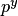that are increasing as a function of the label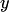, assumed to be a real number. The function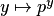is then called a predictive distribution.

A wide class of conformity measures that often lead to conformal predictive systems is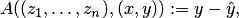where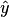is the prediction for the label of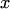based on the training sequence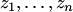and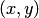. An even wider class is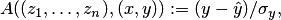where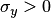is an estimate of the variability or difficulty ofcomputed from the training sequence and. (The methods for computingand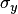are supposed invariant with respect to permutations of.) The width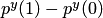of such conformal predictive distributions is typically equal to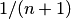, where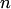is the length of the training sequence, except for at mostvalues of.

The formal definition of conformal predictive systems takes account of the fact that, in the case of smoothed conformal predictors,also depends on the random number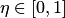, and a fuller notation is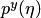. It is also required that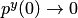as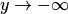and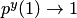as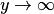.

Notice that in the context of conformal predictive systems the p-values acquire properties of probabilities. Besides, they have some weak properties of object conditionality: e.g., the central prediction regions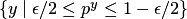are not empty, except in very pathological cases.

There are universally consistent predictive distributions (Vovk, 2017).

## Conformal decision making

Conformal predictive systems can be applied for the purpose of decision making. Universally consistent predictive distributions can be used for making asymptotically efficient decisions.

Bibliography